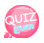# Exit the loop - break

If the loop works and you meet certain conditions, you can stop the loop.
Loops lose their function.

## how to use break statement

So let's look at an example.
The following is an example in which the for statement works and immediately encounters a break statement and loses its functionality.

```<?php
for(\$i = 0; \$i <= 10; \$i++){
break;
echo \$i.'<br>';
}
?>```

So when I check the result there is no output.
Then let's meet the break statement under certain conditions.
In the example above, if the value of variable i is 6, let's exit the for loop.

```<?php
for(\$i = 0; \$i <= 10; \$i++){
echo \$i.'<br>';
if(\$i == 6) break;
}
?>```

Here is the resulting imageShould we also do it in the while statement?

```<?php
\$i = 0;
\$max = 10;

while(\$i <= \$max){
echo \$i.'<br>';
if(\$i == 6) break;
\$i++;
}
?>```

Here is the resulting image